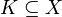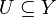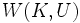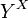# Compact-open topology

This article defines a function space topology i.e. a topology on the collection of continuous maps between two topological spaces

## Definition

Suppose$X$ and$Y$ are topological spaces. The compact-open topology is a topology we can define on the space of continuous functions$C(X,Y)$ from$X$ to$Y$ as follows.

For a compact subset$K \subseteq X$ and an open subset$U \subseteq Y$, we define$W(K,U)$ as the set of all continuous maps$f:X \to Y$ such that$f(K) \subseteq U$. The compact-open topology is the topology with subbasis as the set of all$W(K,U)$s.

## Relation with other function space topologies

Topology Meaning Relationship with compact-open topology
topology of pointwise convergence topology chosen such that a sequence of functions converges iff it converges pointwise; equivalently, the subspace topology inherited from the product topology on the space$Y^X$ of all functions.  ?
topology of uniform convergence
topology of compact convergence

## Particular cases

Case for$X$ Case for$Y$ Overall conclusion Necessarily equals topology of pointwise convergence? Necessarily equals topology of uniform convergence? (if that makes sense)
one-point space anything canonically identified with$Y$, identification is a homeomorphism Yes Yes
discrete space anything canonically identified with$Y^X$, space of all functions from$X$ to$Y$, with the product topology Yes Yes (if that makes sense)
anything T0 space also a T0 space: see function space to T0 space is T0 under compact-open topology No No
anything T1 space also a T1 space: see function space to T1 space is T1 under compact-open topology No No
anything Hausdorff space also a Hausdorff space: see function space to Hausdorff space is Hausdorff under compact-open topology
anything regular space also a regular space: see function space to regular space is regular under compact-open topology
anything completely regular space also a completely regular space: see function space to completely regular space is completely regular under compact-open topology